# DAV Class 5 Maths Chapter 7 Worksheet 3 Solutions

The DAV Maths Book Class 5 Solutions and DAV Class 5 Maths Chapter 7 Worksheet 3 Solutions of Multiplication and Division of Decimal Numbers offer comprehensive answers to textbook questions.

## DAV Class 5 Maths Ch 7 Worksheet 3 Solutions

Question 1.
Find the product orally.
(a) 0.2 × 10
Solution:
0.2 × 10 = 2.0
(Decimal point shift one place to right)

(b) 1.18 × 10
Solution:
1.18 × 10 = 11.8
(Decimal point shift one place to right)

(c) 13.293 × 10
Solution:
13.293 × 10 = 132.93
(Decimal point shift one place to right)(d) 16.25 × 100
Solution:
16.25 × 100 = 1625
(Decimal point shift two places to right)

(e) 4.02 × 100
Solution:
4.02 × 100 = 402
(Decimal point shift two places to right)

(f) 19.32 × 100
Solution:
19.32 × 100 = 1932
(Decimal point shift two places to right)

(g) 71.821 × 1000
Solution:
71.821 × 1000 = 71821
(Decimal point shift three places to right)

(h) 45.01 × 1000
Solution:
45.01 × 1000 = 45010
(Decimal point shift three places to right)

(i) 0.1 × 100
Solution:
0.1 × 100 = 10
(Decimal point shift two places to right)

(j) 7.538 × 100
Solution:
7.538 × 100 = 753.8
(Decimal point shift two places to right)Question 2.
Complete the following:
(a)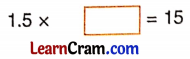Solution:
1.5 × 10 = 15

(b)Solution:
2.61 × 100 = 261

(c)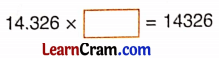Solution:
14.326 × 1000 = 14326

(d)Solution:
0.8 × 10 = 80(e)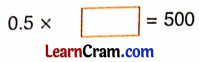Solution:
0.5 × 1000 = 500

(f)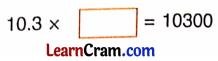Solution:
10.3 × 1000 = 10300

(g)Solution:
3.08 × 10 = 30.8

(h)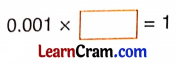Solution:
0.001 × 1000 = 1

DAV Class 5 Maths Chapter 7 Worksheet 3 Notes

In order to multiply a decimal number by 10, 100, or 1000 we just shift the decimal point in the product to the right by as many places as there are zeroes in the multiplier.

Example 1.
Multiply 7.32 x 10
Solution:
7.32 × 10 = 73.2
(Decimal point shift one place to the right)Example 2.
Multiply 5.781 × 100
Solution:
5.781 × 100 = 578.1
(Decimal point shift two places to the right)

Example 3.
Multiply 19.356 × 1000
Solution:
19.356 × 1000 = 19356
(Decimal point shift three places to the right)